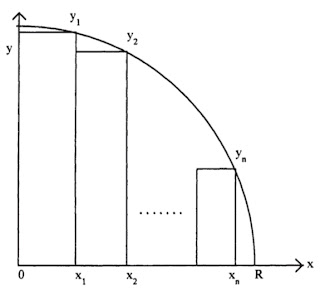### Stacked and Wound Core Construction

1. Introduction
In both core-form and shell-form types of construction, the core is made of thin layers or laminations of electrical steel, especially developed for its good magnetic properties. The magnetic properties are best along the rolling direction so this is the direction the flux should naturally want to take in a good core design. The laminations can be wrapped around the coils or stacked.
The laminations for both types of cores are coated with an insulating coating to prevent large eddy current paths from developing which would lead to high losses.

2. Wrapped or wound cores :
- Wrapped or wound cores have few, if any, joints so they carry flux nearly uninterrupted by gaps.
- the core is wound into a continuous "coil". The core is then cut so that it can be inserted around the coils.
- The cut laminations are then shifted relative to each other and reassembled to form a staggered stepped type of joint. This type of joint allows the flux to make a smoother transition over the cut region than would be possible with a butt type of joint where the laminations are not staggered.
- Very often, in addition to cutting, the core is reshaped into a rectangular shape to provide a tighter fit around the coils. Because the reshaping and cutting operations introduce stress into the steel which is generally bad for the magnetic properties, these cores need to be reannealed before use to help restore these properties.
- A wound core without a joint would need to be wound around the coils or the coils would need to be wound around the core. Techniques for doing this are available but somewhat costly.

Application  :
in small distribution transformers, wound cores predominate.
3. Stacked cores :
- Stacked cores have gaps at the corners where the core steel changes direction. This results in poorer magnetic characteristics than for wound cores.
- the coils are circular cylinders which surround the core. Therefore the preferred cross-section of the core is circular since this will maximize the flux earning area.
- In practice, the core is stacked in steps which approximates a circular cross-section as shown in Fig. 2.
- Note that the laminations are coming out of the paper and carry flux in this direction which is the sheet rolling direction. The space between the core and innermost coil is needed to provide insulation clearance for the voltage difference between the winding and the core which is at ground potential. It is also used for structural elements.Fig.  2  Stepped core used in core-form transformers to approximate a circular cross-section
For a given number of steps, one can maximize the core area to obtain an optimal stacking pattern. Fig. 3 shows the geometric parameters which can be used in such an optimization, namely the x and y coordinates of the stack corners which touch the circle of radius R. Only 1/4 of the geometry is modeled due to symmetry considerations.Fig.  3  Geometric parameters for finding the optimum step pattern

The corner coordinates must satisfy
................(1)
For a core with n steps, where n refers to the number of stacks in half the core cross-section, the core area, An, is given by
...........(2)
where Xo=0. Thus the independent variables are the xi since the yi can be determined from them using (1) To maximize An, we need to solve the n equations
.........(3)
We can show that

.........(4)
so that the solution to (3) does represent a maximum. Inserting (2) into (3), we get after some algebraic manipulation,
.........(5)
In the first and last equations (i = 1 and i = n), we need to use Xo=0 and Xn+1=R.

Since (5) represents a set of non-linear equations, an approximate solution scheme such as a Newton-Raphson iteration can be used to solve them. Note that these equations can be normalized by dividing by R4 so that the normalized solution coordinates xi/R are independent of R. Table 1.1 gives the normalized solution for various numbers of steps.

In practice, because only a limited number of standard sheet widths are kept in inventory and because stack heights are also descretized, at least by the thickness of an individual sheet, it is not possible to achieve the ideal coverage given in the table. Fig. 4 shows a 3 phase stepped core for a core-form transformer without the top yoke. This is added after the coils are inserted over the legs. The bands around the legs are made of a high strength non-conducting material. They help hold the laminations together and prevent them from vibrating in service. Such vibrations are a source of noise.

Application :
In larger power transformers, stacked cores are much more common